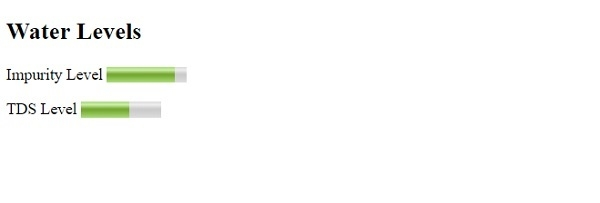# HTML max Attribute

The max attribute of the <meter> element is used to set the upper bound for <meter>. However, the <meter> element is used to measure data with a give range like water impurity level and it is set using the min and max attribute.

## Syntax

Following is the syntax −

<meter max="num">

The num above is a number in floating-point that sets the max attribute of the <meter> element.

## Example

Let us now see an example to implement the max attribute of the <meter> element −

Live Demo

<!DOCTYPE html>
<html>
<body>
<h2>Water Levels</h2>
<p>Impurity Level <meter min="0" low="50" high="95" max="100" value="85"></meter></p>
<p>TDS Level <meter min="0" low="20" high="80" max="100" value="60"></meter></p>
</body>
</html>

## Output

This will produce the following output −In the above example, we have displayed meter for water levels using the <meter>. For maximum value of the meter, we have used the max attribute −

TDS Level <meter min="0" low="20" high="80" max="100" value="60"></meter>